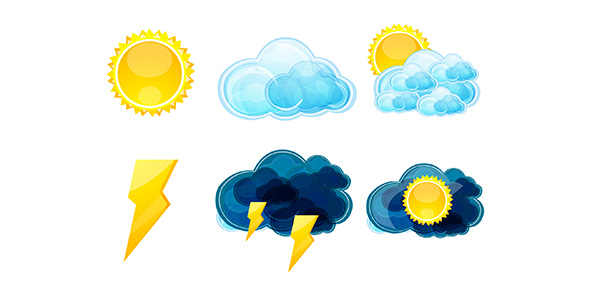# Take This Practice Quiz On Weather!

10 Questions | Total Attempts: 2680SettingsThis quiz has been created for students to ascertain the essential weather changes which can be determined in terms of temperature, humidity, cloudiness, visibility, brightness, wind, and atmospheric pressure, as well as climate changes. Just go through the questions and choose the correct answers. So, let's try out the quiz. All the best!

• 1.
Air pressure is...
• A.

The amount of mass in a unit of volume.

• B.

The result of the weight of a column of air pushing down on an area

• C.

Any instrument that measures changes in pressure.

• D.

The state of the atmosphere at any particular time.

• 2.
A barometer is...
• A.

The amount of mass in a unit of volume.

• B.

An instrument that measures wind speed and direction.

• C.

An instrument that measures air pressure.

• D.

Someone who studies weather.

• 3.
Altitude is...
• A.

The amount of mass in a unit of volume.

• B.

An amount of space.

• C.

The state of the atmosphere at any particular time.

• D.

Distance above sea level; elevation.

• 4.
As altitude increases, air density...
• A.

Increases.

• B.

Decreases.

• 5.
Calculate the density when mass is 100g and volume is 25mL.
• A.

2500 mL/g

• B.

2500 g/mL

• C.

0.25 g/mL

• D.

4 g/mL

• 6.
Calculate the volume if mass is 50 lbs and density is 5 lbs/mL.
• A.

250 mL

• B.

250 lbs

• C.

10 mL

• D.

0.10 lbs

• 7.
What is one human activity that causes large amounts of soil particles to enter the atmosphere.
• A.

Farming

• B.

Waves

• C.

Smoking

• D.

Burning fossil fuels

• 8.
What is a health effect of air pollution?
• A.

Asthma

• B.

Cough

• C.

Chest pains

• D.

All of the above

• 9.
The range between the daily maximum temperature and the daily minimum temperature is usually greater if the air is:
• A.

Very dry

• B.

Very humid

• C.

Neither dry nor humid

• D.

None

• 10.
Which cloud often has an anvil-shaped top?
• A.

Cumulonimbus

• B.

Cirrus

• C.

Cumulus

• D.

Stratus

Related TopicsBack to top